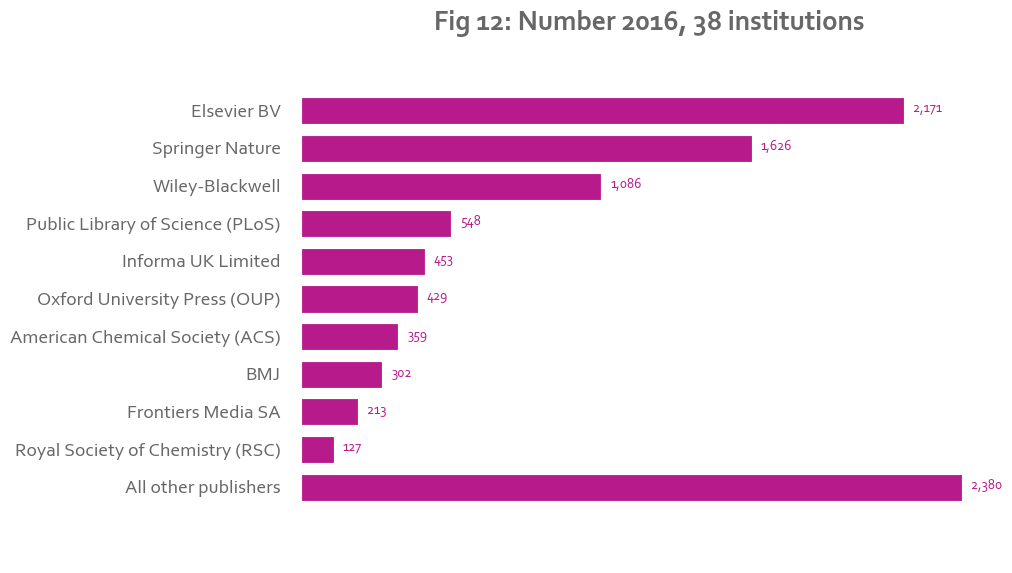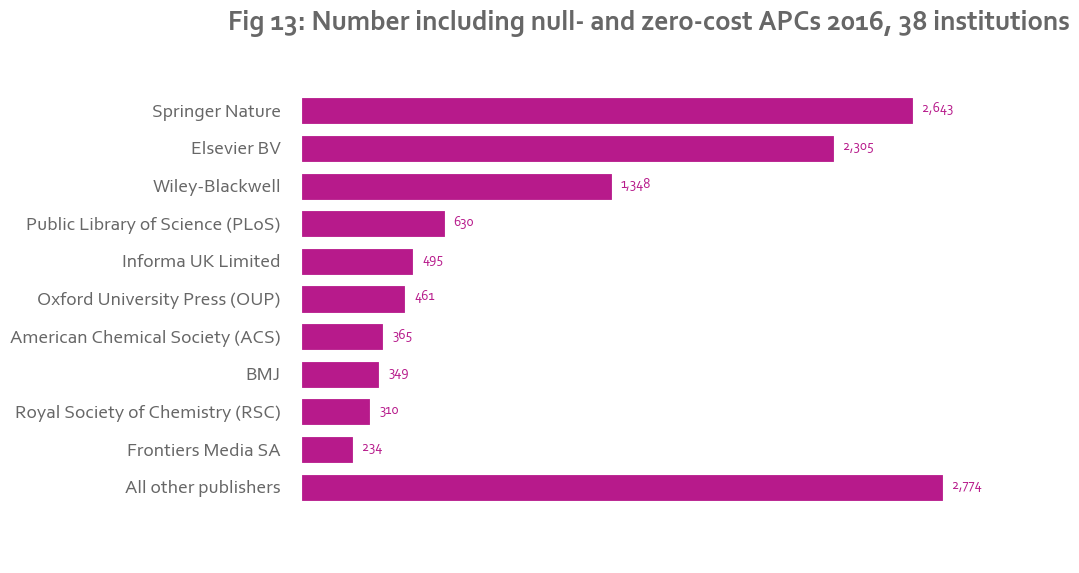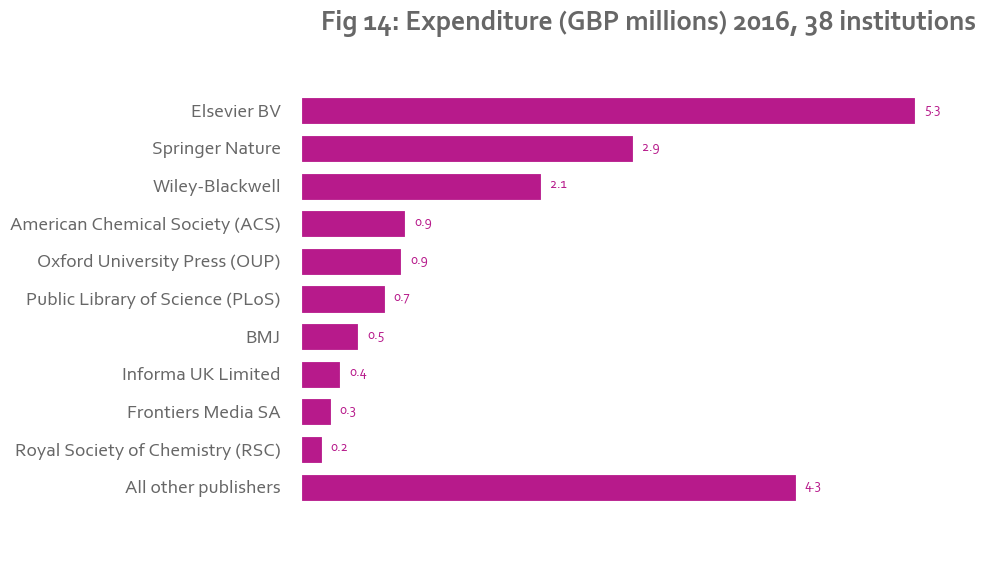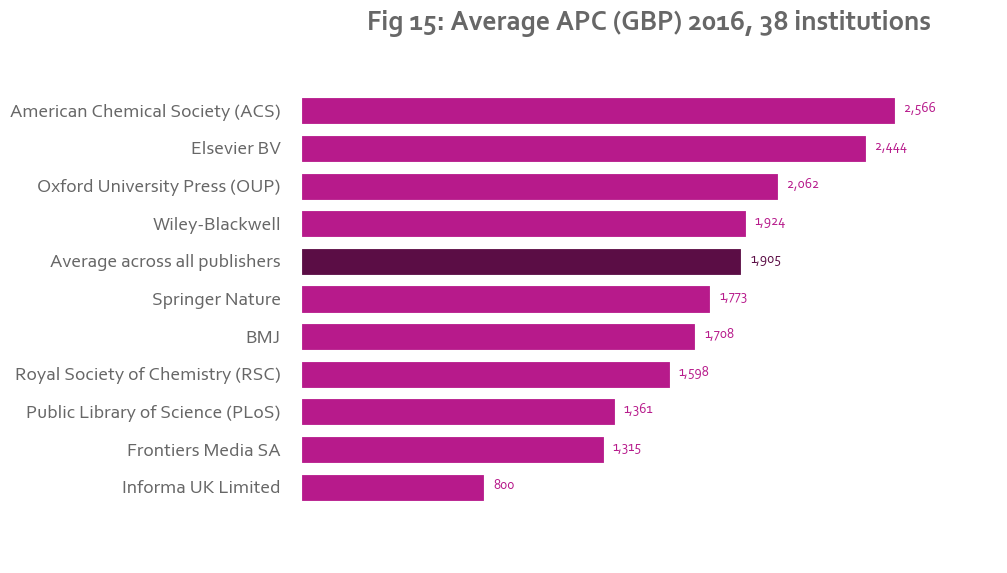# Setup¶

The text and graphs in this report are generated in Python. This notebook looks best on NBViewer.

The data can also be found on Figshare.

In :
from IPython.display import HTML
HTML('''<script>
code_show=true;
function code_toggle() {
if (code_show){
$('div.input').hide(); } else {$('div.input').show();
}
code_show = !code_show
}
</script>
The raw code for this IPython notebook is hidden by default for easier reading.
<a href="javascript:code_toggle()">Toggle on/off the raw code</a>.''')

Out:
The raw code for this IPython notebook is hidden by default for easier reading. Toggle on/off the raw code.
In :
%matplotlib inline

import pandas as pd
import numpy as np
import os
import math
import matplotlib
import matplotlib.mlab as mlab
import matplotlib.pyplot as plt
from matplotlib import style
import itertools
from collections import OrderedDict
from matplotlib.ticker import Formatter
import scipy as sp
import scipy.stats


## Setting the variables¶

The costs in all the analyses below are from the "APC paid (£) including VAT (calculated)" column. For more information about how this is calculated, see the notes on the data clean below.

All analyses by year rely on the "Year expense incurred" column. For more information about what this is, see below.

In :
# Years
start_year = 2013
current_year = 2016
end_year = current_year

years = map(str, range(start_year, end_year+1))

top = 10 # Number of publishers we want to look at as the top publishers

apc_col = u"APC paid (£) including VAT (calculated)" # save having to type it
year_col = "Year expense incurred"

type_of_pubs = ["Journal Article/Review", "Conference Paper/Proceeding/Abstract"]

# Inputs
folder = ""
apc_files = [ year + ".xlsx" for year in years ]

# Outputs
data_folder = r"Processed data"
viz_folder = r"Visualizations"

png_directory = os.path.join(folder, viz_folder, "PNGs")
svg_directory = os.path.join(folder, viz_folder, "SVGs")
data_directory = os.path.join(folder, data_folder)

for directory in [png_directory, svg_directory, data_directory]:
if not os.path.exists(directory):
os.makedirs(directory)
data_file = os.path.join(data_directory, r"Data.xlsx")


## Defining useful functions¶

### Data processing¶

The key values that will be calculated in this analysis are:

• Number,
• Expenditure (GBP),
• Average APC (GBP),
• Median APC (GBP),
• Number including null- and zero-cost APCs

• Number (thousands), and
• Expenditure (GBP millions)

are calculated to make plotting easier.

They are calculated as follows:

• Number : The number of APCs that have cost data associated with them. This number does not include APCs entered at £0 or APCs where the APC paid field is blank.
• Expenditure (GBP): The sum of the APC costs including VAT.
• Average APC (GBP): The mean APC, calculated excluding £0 APCs.
• Average APC (GBP) 95% confidence interval (lower bound): Calculated as
• Average APC (GBP) 95% confidence interval (upper bound):
• Median APC (GBP): The median APC, calculated excluding £0 APCs.
• Number including null- and zero-cost APCs: The number of APCs including those where the APC paid is blank or is £0.

The reasons why null- and zero-cost APCs were not included in calculating number, average APCs, and median APCs will be discussed below.

### Separating the top values¶

There are some cases where for clarity, it is useful to look at a subset of data and group the rest together under the category "Other". For example, in some of the analysis below the top-earning publishers by overall expenditure are shown individually, while the rest are grouped under the category "Other".

### Growth¶

Growth is calculated as a percentage and is defined as (current-old)/old.

In :
def mean_confidence_interval(data, confidence=0.95):
a = 1.0*np.array(data)
n = len(a)
if n > 1:
mean = np.mean(a)
se = scipy.stats.sem(a)
h = se * sp.stats.t.ppf((1+confidence)/2., n-1)
return (mean-h, mean+h)
else:
return [np.nan,  np.nan ]

def process_df(df_w_zeros, groupby=None):

"""
Process a data frame of APC data to get key values for the overall DataFrame or, if groupby is specified, for each group.

Parameters:
df_w_zeros : A data frame of APC data including £0 and null APCs
groupby: list of columns to group by, or None to process the whole data frame.

Returns:
A dataframe with the columns:
Number,
Number (thousands),
Expenditure (GBP),
Expenditure (GBP millions),
Average APC (GBP),
Average APC (GBP) 95% confidence interval (lower bound),
Average APC (GBP) 95% confidence interval (upper bound),
Median APC (GBP),
Number including null- and zero-cost APCs
for the data frame passed overall, or, if groupby is specified, with the values for each group.

Number, Number (thousands), Average APC (GBP), and Median APC (GBP) are calculated excluding £0 and null APCs
"""
# Get the non-zero APCs only
nonzero = df_w_zeros.loc[df_w_zeros[apc_col] > 0]
if groupby:
nonzero = nonzero.groupby(groupby) #group by the specified columns
number = nonzero.size() # Get cut_top(the size of the groups
expenditure = nonzero.agg(np.sum)[apc_col] # get the sum of the groups
mean = nonzero.agg(np.mean)[apc_col] # get the mean of the groups not including £0 APCs
confidence_interval_lower = nonzero.agg(lambda x: mean_confidence_interval(x, confidence=0.95))[apc_col]
confidence_interval_upper = nonzero.agg(lambda x: mean_confidence_interval(x, confidence=0.95))[apc_col]
median = nonzero.agg(np.median)[apc_col] # get the median  of the groups not including £0 APCs
number_w_zero = df_w_zeros.groupby(groupby).size() # get the size of the groups if £0 APCs are included
else:
number = len(nonzero) # the number of non-zero APCs
expenditure = nonzero.sum()[apc_col] # the sum of the APCs
mean= nonzero.mean()[apc_col] # the mean not including £0 APC
confidence_interval_lower, confidence_interval_upper = mean_confidence_interval(nonzero[apc_col])
median = nonzero.median()[apc_col]
number_w_zero = len(df_w_zeros)# the number including £0 APCs
dct = {
"Number" : number,
"Number (thousands)" : number / 1000,
"Expenditure (GBP)" : expenditure,
"Expenditure (GBP millions)" : expenditure / 1000000,
"Average APC (GBP)" : mean,
"Average APC (GBP) 95% confidence interval (lower bound)" : confidence_interval_lower,
"Average APC (GBP) 95% confidence interval (upper bound)" : confidence_interval_upper,
"Median APC (GBP)" : median,
"Number including null- and zero-cost APCs" : number_w_zero
}
# Need to set the index if there is only one row
if groupby:
index = number_w_zero.index
else:
index = 
return pd.DataFrame(dct, index=index)

# This is a function to process the df, separating out values in a specified column
# So for example, to get a df with rows for the top 10 publishers
# plus a row with "Other" that has all other publishers + a row with "Total" that has the total aross all publishers
# Give it a DF including zero values, the name of the column you want to separate by,
# and the name of the values in the column you want to keep
# Optionally add a list of other columns to group by

def cut_top(df_w_zeros, col, lst, other_groupby=None):
"""
Return a processed Data Frame with a row for each value specified in list, a row with all other values processed together, and a total row.

Parameters:
df_w_zeros : A data frame of APC data including £0 and null APCs
col: The column in which to look for the specified values
lst: A list of the values to separate
other_groupby: List of other columns to group by (not including col), or None.

Returns:
A DataFrame with the following columns:
Number,
Number (thousands),
Expenditure (GBP),
Expenditure (GBP millions),
Average APC (GBP),
Median APC (GBP),
Number including null- and zero-cost APCs
and a row (or rows if grouped) for each item in lst, as well as an 'Other' and 'Total' row
"""
# Group by the given column by default, then any additional columns specified
if other_groupby:
if not isinstance(other_groupby, list):
other_groupby = [other_groupby]
groupby = [col] + other_groupby
else:
groupby=col
# Separate out the given values and the other values
top = df_w_zeros.loc[df_w_zeros[col].isin(lst)]
other = df_w_zeros.loc[~df_w_zeros[col].isin(lst)]

# Process the values
topdf = process_df(top, groupby).reset_index()
otherdf = process_df(other, other_groupby).reset_index()# Only group by the other_groupby values
totaldf = process_df(df_w_zeros, other_groupby).reset_index() # Only group by the other_groupby values

# Set the names
otherdf[col] = "Other"
totaldf[col] = "Total"

# Concatenate and return a dataframe
df = pd.concat([topdf, otherdf, totaldf])
if "index" in df.columns:
del df["index"]
return df

def growth(series):
""" Calculates percentage growth for a Series"""
curr = series # The current  set
old = series.shift(1) # Shifts all  values down one row
growth = (curr - old)/old
return growth


### Formatting¶

Some of the text is generated directly from the code below. Formatting functions are defined to make these easier to read.

Numbers are formatted to 0 or 1 decimal places as appropriate. Percentages are shown out of 100 and are rounded to the nearest whole number. Percent growth is shown out of 100 and prepended with a "+" if growth is positive. Lists are separated by commas with "and" before the last value.

In :
# Formats number as a number with n decimal places
def numf(x, n):
"""Format a number x with n decimal places"""
return "{0:,.{1}f}".format(x, n)

def pctf(x):
""" Format a number as a percentage with no decimal"""
return "{0:.0f}".format(x*100) + "%"

# formats number as growth with a + and percentage sign
def growthf(x):
"""Format a number as a percentage growth with a + sign if positive"""
if pd.isnull(x):
return ""
else:
if x > 0:
return "+" + pctf(x)
return pctf(x)

def printlist(lst):
"""
Print list as string of items separated by commas and with "and" before the last item

Examples:
printlist(["One"]) returns "One"
printlist(["One", "two"]) returns "One and two"
printlist(["One", "two", "three"]) returns "One, two, and three"
"""
if len(lst) == 1:
return lst
if len(lst) == 2:
return lst + " and " + lst
lst = [item + "," for item in lst[0:len(lst) - 1]] + [lst[len(lst)-1]]
lst[len(lst) - 2] = lst[len(lst) - 2] + " and"
return " ".join(lst)


### Saving¶

As the report runs, the figures are saved as PNGs and SVGs with a name format such as "00 - figure title.png".

The data behind the figures is saved to an Excel workbook where the sheet name denotes the figure number(s) for which the data is used

In :
fig_num = 1
next_fig_to_save = fig_num

# Save as PNG and SVG to folder and increment figure number
def save_plot(title):
"""
Save a plot as PNG and SVG to the directories specified above.

Parameters:
title: the title of the plot

The filename will have the format '00 - title.ext'
"""
global fig_num
figname = "{:02d} {}.png".format(fig_num, title)
plt.savefig(os.path.join(png_directory, figname), bbox_inches="tight")
figname = "{:02d} {}.svg".format(fig_num, title)
plt.savefig(os.path.join(svg_directory, figname), bbox_inches="tight")
fig_num += 1

writer = pd.ExcelWriter(data_file, engine='xlsxwriter')

# Save DF as a new sheet in an Excel workbook
def save_df(df, **kwargs):
"""
Add a data frame as a new sheet in an ExcelWriter object.

Parameters:
df: data frame to save
kwargs: additional arguments to pass to pd.to_excel()

The sheet will have a name like 01-04 indicating which figures it is the underlying data for
"""
global fig_num, next_fig_to_save
global writer
if next_fig_to_save == fig_num - 1:
sheet_name = "{:02d}".format(next_fig_to_save)
else:
sheet_name = "{:02d} - {:02d}".format(next_fig_to_save, fig_num-1)
df.to_excel(writer, sheet_name=sheet_name, **kwargs)
next_fig_to_save = fig_num



The data is stored in a separate file for each year of collection. It is merged to form a master data set.

In :
# So, eg, '2016' will be read as a string not a float
converters={
'Date of initial application by author' : str,
'Date of publication':str,
'Date of APC payment':str
}

df_list = []
for f in apc_files:
temp_df.loc[temp_df['Public?']].to_excel(os.path.join(folder, f.replace(".xlsx", " - public.xlsx")), index=False)
temp_df["TCO year"]  = f.replace(".xlsx", "")
df_list.append(temp_df)
master_df = pd.concat(df_list)


# Cleaning the data¶

The Jisc Total Cost of Ownership project (TCO) began in 2013. The project asks higher education institutions to provide data on their spending on article processing charges. The data is collected in a standardized format and is made openly available.

The data is collected on a yearly basis for the calendar year.

Since its inception in 2013, the TCO data format has changed several times. The 2013 data set has fewer columns than in the following years. The 2014-2016 templates are similar and contain 39 columns with which to record bibliographic, funder, payment, licence, and other information. Next year, 2017, the template has been changed again. The columns have been pared down to reduce reporting burden on institutions. In particular, institutions have been instructed that they do not need to provide bibliographic data (Journal, ISSN, Publisher, and Type of publication) if they provide a DOI or PubMed ID, since these IDs can be queried against the Crossref or PubMed API. The values that can be entered in the "Fund that APC is paid from," "Funder of research," "Type of publication," and "Licence" columns have been constrained in order to standardise how data was collected. Finally, a new sheet has been added to help institutions calculate the value of APCs paid for from discount, membership, and pre-payment deals.

Although none of the data has been entered in the new TCO format, I have cleaned the data from past years to bring it into line with the new format whenever possible, so it will be compatible with data entered in the new format going forwards.

## Data clean method¶

### Cleaning data on OpenRefine¶

The data was cleaned on OpenRefine, a tool for cleaning messy data.

### The data set¶

The data set contains two sets of columns. The columns ending with "(old)" are the original values as entered by the institution. "TCO year" indicates which year the institution submitted the data to Jisc.

### Data formats and values¶

All dates are in YYYY-MM-dd, YYYY-MM, or YYYY format.

Publisher names have been changed as much as possible to match the publisher's name in Crossref.

"Fund that APC is paid from," "Funder of research," "Type of publication," and "Licence" have been standardised to match a constrained list of values.

"Currency of APC" is in three-letter format (eg GBP, EUR).

DOIs, PubMed IDs, and PubMed Central IDs have been cleaned to the appropriate format.

### Data from external sources¶

The data has been cleaned to use a standard set of terms where possible. It has been augmented with data from the Crossref, Pubmed, and Pubmed Central APIs. It has also been enhanced with data from DOAJ and exchange rates from Fixer.io and the European Central Bank.

In the case of bibliographic information (Journal, ISSN, Publisher, and Type of publication), data from external sources takes preference. The data from these sources is reliable, complete, and more standardised than that entered by hand. The article title provided by the institution was checked against the article title found in the external source. In the case of discrepancy between the two, the data from the external source was discarded.

For all other fields, data provided by the institution takes preference, and external sources are used only when the data is missing.

New columns have also been created using external data. "Journal type" indicates whether the journal is hybrid or full open access based on whether the ISSN can be found in DOAJ. "Exchange rate" is the exchange rate for the given currency on the date of payment using the Fixer.io API, or the average exchange rate for that year if the date of payment is not provided.

In the first year of TCO, 2013, institutions were not asked to provide a DOI, PMID, PMCID, or ISSN. Therefore data for this year has not been supplemented from external sources.

### Calculated values¶

"Journal type" indicates whether either of the journal's ISSNs can be found in DOAJ. The journal type is "Full OA" if the ISSN is in DOAJ, "Hybrid" if it is not, and blank if there is no ISSN.

"Exchange rate" is the exchange rate from the "Currency of APC" into pounds on the date of payment if the "Date of payment" column has a full date. If not, the exchange rate is the yearly average exchange rate given by the European Central Bank.

"APC paid (£) including VAT (calculated)" is the "APC paid (£) including VAT if charged" from the institution if this is available. If not, it is calculated by multiplying the "APC paid (actual currency) including VAT if charged" column by the "Exchange rate" column.

"Year expense incurred" is calculated by taking highest value between the year in "Date of publication" and "Date of APC payment." In most cases, the APC is paid before the article is published. The date of publication therefore represents the date that the publisher has fulfilled its obligation to make the article open access. Until this point, the institution must account for the cost of the APC as a credit with the publisher; after this date, they can incur it as an expense. In the case where they chose to make the article open access retrospectively, the expense will be incurred on the date the article is made open access. In this case, the date of APC payment is used as a proxy for the date the article is made OA.

### De-duplication¶

The data was de-duplicated along the DOI, PMID, PMCID, and article title. The "Duplicate?" column is "True" if the row has been identified as a duplicate.

For each group of duplicated entries, I identified which rows to drop as follows:

• If one or more of the entries have an APC cost, keep the one(s) with the cost. If they all have a cost or all have null or £0 entries, keep all.
• If the entries were submitted in different years, keep the one(s) from the most recent year, as we assume this has the most up-to-date information.
• If the entries are from the same institution in the same year, and have the same cost, keep the one that has the most columns filled out (or if equal, drop either one). This assumes that the institution has recorded the APC twice by accident rather than having paid it twice.

The remaining duplicates will have different costs and/or were entered by different institutions. In this case, there is no way to know which is correct, and all were left in the data set.

The column "Drop?" is True if the duplicates should be dropped according to the method above.

## Issues with the data¶

### Lack of standardization¶

As mentioned above, the data has for the most part been entered by hand by members of the institutions. As such, especially over a large data set, there is a large variation in the way the data has been entered. I have tried to be consistent in bringing this data into a standardized format, but inevitably, some choices are editorial.

### Quality and timeliness of data from external sources¶

The data is supplemented from external sources. This greatly enriches the data and provides fuller and more accurate information than would be available otherwise. However, the datasets used are accurate to the time they were called, and do not represent the situation as it was in the past. For example, journals that have been acquired by a new publisher over the TCO period will be listed under the new publisher rather the one who owned them at the time the APC was paid. Likewise, a hybrid journal might be listed as full OA if it flipped during the span of TCO.

The case of Springer and Nature Publishing Group makes this most apparent. These two publishers merged in 2015 to form Springer Nature. In this analysis, in the years 2013-2016 Springer and Nature are considered together as Springer Nature to make comparisons easier.

### Discount, membership, and pre-paid APCs¶

In the case of discount, membership, and pre-paid APCs, the cost of the APC can be unclear. In many cases the users of the spreadsheet entered these without a value, recorded them at £0, or simply did not record them at all. The next iteration of the TCO spreadsheet will provide a way for institutions to estimate the costs of these APCs. However, there is currently no way to estimate the true costs of these APCs or even how many of them have been paid. In the case of Springer Nature, for example, we see a high occurence of £0 APCs and can assume that most of these refer to offset APCs. However, for Springer Nature APCs that do have a cost associated, there is no way of knowing how many refer to offest APCs.

For the purpose of this analysis, £0 APCs have been excluded when calculating the average and median APC. This is because in most cases, the £0 APC indicates that the cost of the APC was not clear, not that there was no cost associated. "Number" refers to the number excluding these £0 APCs, and "Number including null- and zero-APCs" refers to the total number.

### Time periods and dates¶

Jisc collects TCO in January for the full calendar year of the preceeding year. RCUK and COAF also use the Jisc template and have different reporting periods. In some cases, an institution may not be able to collect data for the full calendar year and will send their RCUK and/or COAF returns instead. Information on this is included in the Notes file link.

In addition, it is not always clear which APCs fall within the period. The template includes three dates: the date of initial application by author, the date of payment, and the date of publication. For this analysis, I have considered an APC to belong to the most recent year between the year of publication and year of APC payment. This year represents the year that, from the institution's perspective, the expense was incurred on the balance sheet, and from the publisher's perspective, the year that the revenue was generated.

### Non-public data¶

Each year Jisc asks to make APC data publicly available. In the past years a handful of institutions have opted out of making their data public and have asked that it be used in aggregate analyses only. These institutions' data is used in the analyses below but has been removed from the public data set.

### Currency fluctuation¶

The year 2016 was a volatile year for the pound. Given the fluctuating price of the pound, APCs calculated from another currency have used the exchange rate of the date of payment wherever this is available. When the pound is weak, APCs paid to publishers abroad will be more expensive once converted. The prices have not been adjusted to reflect the change in the value of the pound since 2013. This would be an interesting topic for further study.

### Duplicates in the data set¶

As mentioned above, even after removing some duplicates, there are still some duplicates in the data set. It is not clear whether these duplicates refer to APCs that have been paid twice, have been split between institutions, or have simply been recorded twice by accident.

In :
master_df = master_df.reset_index() # Use the "index" column to know which DOIs to keep/delete
master_df["Duplicate?"] = False
master_df["Drop?"] = False
dropped_rows = []
for identifier in ["DOI", "PubMed ID", "PubMed Central (PMC) ID", "Article title"]:
# Don't consider rows that were already dropped on previous passes
df = master_df.loc[~master_df["Drop?"]]
duplicates = df[identifier].loc[df.duplicated(identifier)].dropna() # Get list of duplicated DOIs without NaN values
master_df.loc[master_df[identifier].isin(duplicates), "Duplicate?"] = True # Identify these as duplicates in the master DF
dup_df = master_df.loc[master_df[identifier].isin(duplicates)]
duplicate_indices_at_start = dup_df["index"].copy()

#---------------------------------------------------------------------------------------------------
# If there are several entries and one or more has a value for the APC paid, keep the ones with an entry
# and drop the ones that don't have an entry for APC paid.
# If all entries have an APC cost or all entries do not, then keep all of them.
#---------------------------------------------------------------------------------------------------
null_or_zero = dup_df[apc_col].fillna(0) == 0 #  Entries where APC is null or zero
dup_df["Null or zero APC?"] = null_or_zero # Create a new column in the DF for APCs that have no entry or a £0 entry. This causes the SettingWithCopyWarning

# Pivot so we have a DF where the rows are DOIs  and the columns are True and False (for Null or zero APC?)
pt = dup_df.pivot_table(index= identifier, columns="Null or zero APC?", values = "index",
aggfunc="count")
# Get a list of the DOIs where there is at least one null entry and at least one non-null entry.
to_drop_null_or_zero = pt.loc[pd.notnull(pt[False]) & pd.notnull(pt[True])].index

# Drop the DOIs that are in that list from dup_df if they have a null value
dup_df = dup_df.loc[~(dup_df[identifier].isin(to_drop_null_or_zero) & dup_df["Null or zero APC?"])]

#---------------------------------------------------------------------------------------------------
# For the remaining entries, keep the one(s) that were submitted in the most recent TCO year,
# assuming that these contain the most accurate data
#---------------------------------------------------------------------------------------------------
# Get a sorted list of the years that DOI appeared in
df= dup_df[[identifier, "TCO year"]].groupby(identifier).agg(lambda x: sorted(list(x.unique())))
# The last year in the list will be the year to keep
df["Year to keep"] = df["TCO year"].apply(lambda x: x[-1])
# Keep only those values
dup_df = dup_df.loc[dup_df.apply(lambda x: x["TCO year"] == df.ix[x[identifier]]["Year to keep"], axis=1)]

#---------------------------------------------------------------------------------------------------
# If the same institution has a more than one entry with the same date of payment and the same payment,
# then delete all but one entry.
# Keep the entry that has the most columns filled out
#---------------------------------------------------------------------------------------------------
# Data frame which will have "True" in the column if there is more than one entry in that column and the entries are the same
df = dup_df.groupby([identifier, "Institution"]).agg( lambda x: len(x) > 1 and len(set(x))==1 )
dups = df.loc[df["Date of APC payment"] & df[apc_col]]
dups = dups.reset_index()
# Separate these entries out from the data frame
duplicate_rows = dup_df.loc[dup_df[identifier].isin(dups[identifier]) & dup_df["Institution"].isin(dups["Institution"])].sort_values(identifier)
# Drop by preference the ones that have the least complete information
# Calculate the number of non-NaN values in each row
duplicate_rows["Count of non-nan values"] = duplicate_rows.count(axis=1)
# Sort from highest to lowest
duplicate_rows = duplicate_rows.sort_values("Count of non-nan values", ascending=False).reset_index(drop=True)
# Get the rows with the highest count
rows_to_keep = duplicate_rows.drop_duplicates(identifier, keep="first")
# Drop all the duplicate rows identified from the data frame
dup_df = dup_df.loc[~dup_df["index"].isin(duplicate_rows["index"])]
# Add back the rows to keep
dup_df = dup_df.append(rows_to_keep)

# Drop all the duplicates identified at the start, with the exception of those that are still in the duplicate DF
indices_to_drop = set(duplicate_indices_at_start).difference(set(dup_df["index"]) )
# Add the dropped rows to a list
dropped_rows += [indices_to_drop]
# Identify these as rows to drop
master_df.loc[master_df["index"].isin(indices_to_drop), "Drop?"] = True
del master_df["index"]

total_num_rows = len(master_df)
num_duplicates = master_df["Duplicate?"].sum()
num_dropped_rows = master_df["Drop?"].sum()

# Check how many duplicates remain
df = master_df.loc[~master_df["Drop?"]]
num_remaining_duplicates = 0
for identifier in ["DOI", "PubMed ID", "PubMed Central (PMC) ID", "Article title"]:
duplicates = df[identifier].loc[df.duplicated(identifier)].dropna()
num_remaining_duplicates += len(duplicates)

HTML(
"""Of the {} records, {} were identified as duplicates.
In total, {} rows that were identified as duplicates were dropped from the data set.
{} duplicates remain that have not been dropped."""
.format(total_num_rows, num_duplicates, num_dropped_rows, num_remaining_duplicates))

C:\Anaconda\lib\site-packages\ipykernel_launcher.py:20: SettingWithCopyWarning:
A value is trying to be set on a copy of a slice from a DataFrame.
Try using .loc[row_indexer,col_indexer] = value instead

See the caveats in the documentation: http://pandas.pydata.org/pandas-docs/stable/indexing.html#indexing-view-versus-copy

Out:
Of the 36217 records, 2443 were identified as duplicates. In total, 3386 rows that were identified as duplicates were dropped from the data set. 312 duplicates remain that have not been dropped.

## Processing the data¶

In :
# Calculate the "Year expense incurred" column - this will be the year that the article was published, or the date of APC payment for articles made OA retrospectively.
# All dates are in format YYYY-MM-dd
master_df["Year of publication"] = master_df["Date of publication"].apply(lambda x: x.split("-") if pd.notnull(x) else None)
master_df["Year of APC payment"] = master_df["Date of APC payment"].apply(lambda x: x.split("-") if pd.notnull(x) else None)
master_df["Year expense incurred"] = master_df[["Year of publication", "Year of APC payment"]].apply(max, axis=1)

public_df = master_df.loc[master_df["Public?"]]
public_df.to_excel(os.path.join(folder , "ALL APC DATA MERGED.xlsx"), index=False)

master_df.to_excel(os.path.join(folder, "ALL APC DATA MERGED - including non-public institutions not for release.xlsx"), index=False) #

# Get rid of the columns that end with (old) (the original uncleaned data)
master_df=master_df[[col for col in master_df.columns if not col.endswith("(old)")]]

# Get the institutions who participated in all 4 TCOs
trend_insts = master_df.groupby(["Institution", "TCO year"]).size().unstack().dropna(axis=0).reset_index()["Institution"]

# Get the institutions who participated in the current TCO year
current_insts = master_df.loc[master_df["TCO year"] == str(current_year)]["Institution"].unique()

# Also get a list of the top publishers by expenditure overall as we will look at these in more detail
top_pubs = master_df.groupby("Publisher").aggregate(sum)[apc_col].nlargest(top).index # Get the top publishers by expenditure overall

# Drop the duplicates identified above
master_df = master_df.loc[~master_df["Drop?"]]

# If looking at trends, we only want records for the institutions who participated in all 4 TCOs
trend_df = master_df.loc[master_df["Institution"].isin(trend_insts)]
trend_df = trend_df.loc[trend_df[year_col].isin(years)] # And only for the TCO years
trend_df = trend_df.loc[trend_df["Type of publication"].isin(type_of_pubs)] # And keep the types of publications we want to look at

# One outlier skews the results quite a lot because it has a large budget that was cut in 2015. We will sometimes look at the data without this outlier
trend_df_without_outlier = trend_df.loc[trend_df['Institution'] != 'UCL']

# If looking at just this year only, we will look at all institutions who participcated in this TCO year
current_df = master_df.loc[master_df["Institution"].isin(current_insts)]
current_df = current_df.loc[current_df[year_col] == str(current_year)] # Only for the current year
current_df = current_df.loc[current_df["Type of publication"].isin(type_of_pubs)] # And keep only the types of publications we want to look at

HTML(
"<p>Jisc has been collecting APC data since {}. The most recent full year for which we have data is {}.".format(start_year, current_year)

+ "<p>For the purpose of this study, we will only look at APCs paid for APCs of type {}.".format(printlist(type_of_pubs)) +

'''<p>All charges referred to are inclusive of VAT. Some have been calculated using the exchange rate on the date of payment,
or the yearly average exchange rate if no date of payment was provided.
The dates refer to the date that the article was published, or the date the APC was paid
for articles made open access retrospectively.'''
+
'''<p>All analyses referring to the current year only uses the full data set from {} institutions.
The institutions who provided data this year are: {}.</p>

<p>All analyses of trends from {}-{} refer to data only from the {} institutions
who participated in all {} years of data collection. These institutions are: {}. </p>

<p>For clarity, analyses of APC expenditure by publisher look at the top {} publishers by expenditure overall
and group together all other publishers in the data set under the category "Other".  The top 10 publishers by expenditure are: {}.

'''.format(len(current_insts),
printlist(sorted(current_insts)),
start_year,
current_year,
len(trend_insts),
len(years),
printlist(sorted(trend_insts)),
len(top_pubs),
printlist(top_pubs)
)
)

Out:

Jisc has been collecting APC data since 2013. The most recent full year for which we have data is 2016.

For the purpose of this study, we will only look at APCs paid for APCs of type Journal Article/Review and Conference Paper/Proceeding/Abstract.

All charges referred to are inclusive of VAT. Some have been calculated using the exchange rate on the date of payment, or the yearly average exchange rate if no date of payment was provided. The dates refer to the date that the article was published, or the date the APC was paid for articles made open access retrospectively.

All analyses referring to the current year only uses the full data set from 38 institutions. The institutions who provided data this year are: Aberystwyth University, Aston University, Bangor University, Cardiff University, Cranfield University, Durham University, Goldsmiths, Imperial College London, Keele University, King's College London, LSE, Lancaster University, Manchester Metropolitan University, Newcastle University, Northumbria University, Plymouth University, Queen Mary, University of London, Queen's University Belfast, Royal Holloway, St George's, University of London, Swansea University, UCL, University of Bath, University of Birmingham, University of Cambridge, University of Exeter, University of Glasgow, University of Hull, University of Liverpool, University of Manchester, University of Nottingham, University of Oxford, University of Reading, University of Sheffield, University of Southampton, University of St Andrews, University of Sussex, and University of York.

All analyses of trends from 2013-2016 refer to data only from the 11 institutions who participated in all 4 years of data collection. These institutions are: Cranfield University, King's College London, Queen Mary, University of London, Royal Holloway, Swansea University, UCL, University of Birmingham, University of Cambridge, University of Glasgow, University of Liverpool, and University of Sussex.

For clarity, analyses of APC expenditure by publisher look at the top 10 publishers by expenditure overall and group together all other publishers in the data set under the category "Other". The top 10 publishers by expenditure are: Elsevier BV, Springer Nature, Wiley-Blackwell, Oxford University Press (OUP), Public Library of Science (PLoS), American Chemical Society (ACS), BMJ, Informa UK Limited, Royal Society of Chemistry (RSC), and Frontiers Media SA.

## Defining graphing functions¶

### Style¶

In :
style.use("jisc")
# Jisc colours
plot_colours = {
"purple" :"#b71a8b",
"blue" : "#0092cb",
"orange" : "#e85e12",
"pink": "#e61554",
"yellow": "#f9b000",
"green" : "#b2bb1c",
"dark purple" : "#5b0d45",
"dark orange" : "#4a1e06",
"dark blue" : "#004a68",
"dark pink" : "#940e37",
"dark yellow" : "#745300",
"dark green" : "#46490b"
}

font_colours = {
"body" : "#2c3841",
"subtle" : "#666666"
}

background_colour = "#e4e9ec"


### Labelling¶

In :
def autolabel(rects, ax, labels=None, above=True, offsetpct=0.01, **kwargs):
"""
Label each bar of a vertical bar chart or histogram.

Parameters:
rects: the rectangles of the chart
ax: the axis of the chart
labels: list of values to label the bars with (of the same length as rects), or None to use the rect height as label
above: True if the label should appear above the bar, or False to appear underneath
offsetpct: amount to offset by as a percentage of the total height
kwargs: other keyword arguments to pass to ax.text()
"""
# Get y-axis height to calculate label position from.
(y_bottom, y_top) = ax.get_ylim()
y_height = y_top - y_bottom

i = 0
for rect in rects:
height = rect.get_height()

# Fraction of axis height taken up by this rectangle
p_height = (height / y_height)

# Place labels above or below
if above:
label_position = height + (y_height * offsetpct)
else:
if p_height < 0.05:
label_position = height + (y_height * 0.01)
else:
label_position = height * offsetpct

#If no label specified, use value as label
if not labels:
label = '{:,.0f}'.format(height)
else:
label = labels[i]

ax.text(rect.get_x() + rect.get_width()/2., label_position,
label,
ha='center', va='bottom', **kwargs)
i += 1

def special_auto_label_h(rects, ax, labels=None, offsetpct=0.01, specialkwargs=None, **kwargs):
"""
Label each bar of a vertical bar chart or histogram. Can specify keyword arguments for each bar.

Parameters:
rects: the rectangles of the chart
ax: the axis of the chart
labels: list of values to label the bars with (of the same length as rects), or None to use the rect width as label
offsetpct: amount to offset by as a percentage of the total height
specialkwargs: list of form [{kwarg0 : value00, kwarg1: value01}, {kwarg0 : value10, kwarg1: value11}] for the individual rects
kwargs: other keyword arguments to pass to ax.text()

"""
# Get y-axis height to calculate label position from.
(x_left, x_right) = ax.get_xlim()
x_width = x_right - x_left

i = 0
for rect in rects:
width = rect.get_width()

# Fraction of axis height taken up by this rectangle
p_width = width / x_width

# Place labels above or below
# If we can fit the label above the column, do that;
# otherwise, put it inside the column.

label_position = width + (x_width * offsetpct)

#If no label specified, use value as label
if not labels:
label = '{:,.0f}'.format(width)
else:
label = labels[i]

if specialkwargs:
kwargs = specialkwargs[i]
else:
kwargs = {}

ax.text(label_position, rect.get_y() + rect.get_height()/2.,
label,
**kwargs)
i += 1

"""Prepend "Fig {}: " to title """
global fig_num
return "Fig {}: {}".format(fig_num, title)


### Histogram¶

In :
# Plots the histogram, saves the data, and saves the figure
def histogram(x, title, xlabel, binwidth=None, xticklabels=None, labels=None):
"""
Generate a histogram, save it, and plot it.

Parameters:
x: the data for the histogram
title: the title of the plot
xlabel: the label of the x-axis
binwidth: the width of the bins, or None to calculate automatically
xticklabels: the labels of the x-axis ticks
labels: the labels of teach bar
"""
plt.close("all")
fig, ax = plt.subplots(figsize=(20,8))

# Plot the data
if binwidth:
start = x.min() - x.min()%binwidth # Start at a multiple of binwidth
bins = np.arange(start, x.max()+binwidth, binwidth)
n, bins, patches = ax.hist(x, bins=bins)
else:
n, bins, patches = ax.hist(x)

# Rotate the tick labels on the x-axis
if not xticklabels:
xticklabels = map(lambda x: numf(x,0), bins)
ax.set_xticks(bins)
ax.set_xticklabels(xticklabels, rotation='vertical', size=14)

# Set the x-axis label

# Set the y-axis label

# Hide the grid
ax.grid(visible=False)

# Hide the ticks and bring the tick labels closer
ax.tick_params(axis='both', which='both', bottom='off', top='off', right='off', left='off', labelleft='off', pad=-5)

# Hide the axes
ax.spines['right'].set_color('none')
ax.spines['top'].set_color('none')
ax.spines['left'].set_color('none')
ax.spines['bottom'].set_color('none')

# Label each bar with its value
autolabel(patches, ax, labels=labels, color = plot_colours["purple"], rotation="vertical", above=True, size=14)

# Save the plot without the title
save_plot(title)

# Save the data frame
save_df(pd.DataFrame({"Frequency":n, "Bin upper bound":bins[1:]}), index=False)

# Set the title
ax.set_title(title, y=1.08)

# Show the plot
plt.show()

return fig,ax


### Dual-axis line and bar chart¶

In :
def line_bar_chart(x, y1, y2, title, x_tick_labels, x_axis_label, y1_label, y2_label, y1_annotation=None, y2_annotation=None, y1_ticks=None, y2_ticks=None):
"""
Generate a dual-axis chart with bars on the first axis and a line on the second axis, save it, and plot it.

Parameters:
x: the x axis values
y1: the y values for the bar chart
y2: the y values for the line chart
title: the title of the plot
x_tick_labels: the labels of the x-axis ticks
x_axis label: a label for the x axis
y1_label: The label of the bar chart axis
y2_label: the label of the line chart axi
y1_annotation: optional list of labels for growth to add the the bar chart
y2_annotation: optional list of labels for growth to add to the line chart
y1_ticks: Optionally set the ticks of the bar chart y axis
y2_ticks: Optionally set the ticks on the line chart y axis
"""
plt.close('all')
fig, ax1 = plt.subplots(figsize=(9,6.8))

rects = ax1.bar(x,
y1,
width=0.45,
color= plot_colours["dark purple"],
align="center",
tick_label= x_tick_labels,
label=y1_label)

ax2 = ax1.twinx() # duplicates the axis
lines = ax2.plot(x,
y2,
color = plot_colours["blue"],
label = y2_label)
ax1.set_xlabel(x_axis_label, ha="center", size=14)
# Set the y-axis labels
ax1.set_ylabel(y1_label,horizontalalignment="center", color=plot_colours["purple"])
ax2.set_ylabel(y2_label,horizontalalignment="center", color=plot_colours["blue"])
# Hide the spines and ticks
ax1.spines['top'].set_color('none')
ax1.tick_params(axis='both', which='both', top='off')
# Set the axes
if y1_ticks:
ax1.yaxis.set_ticks(y1_ticks)
else:
start, end = ax1.get_ylim()
ax1.yaxis.set_ticks(np.arange(start, end, 1))
if y2_ticks:
ax2.yaxis.set_ticks(y2_ticks)
else:
start, end = ax2.get_ylim()
ax2.yaxis.set_ticks(np.arange(start, end, 1))
# Hide the grid
plt.Axes.grid(ax1, visible=False)
plt.Axes.grid(ax2, visible=False)

if y1_annotation:
autolabel(rects, ax1, labels=y1_annotation, above=False, offsetpct=0.4, color="w", size=12, weight="bold")
# Annotate all with an arrow, except the first one
if y2_annotation:
for X,Y,Z in zip(x[1:], list(y2)[1:], list(y2_annotation)[1:]): # Leave out the first value so the arrow won't show
ax2.annotate(Z, xy=(X,Y), xytext=(-40,15), ha="right", textcoords='offset points', color=plot_colours["blue"], size=12, weight="bold",
arrowprops = dict(arrowstyle='-', linewidth=1, color=font_colours["subtle"]))

p = [rects, lines]
ax1.legend(p, [p_.get_label() for p_ in p], loc="lower left",  bbox_to_anchor=(0, -.302, 0,0),  ncol=2, borderaxespad=2, frameon=False, )
save_plot(title)

ax1.set_title(title, y=1.08)
plt.show()
return fig, ax1, ax2


### Bar chart¶

In :
def bar_chart(x, y, title, x_tick_labels, x_axis_label=None, y_axis_label=None, labels=None):
"""
Generate a bar chart, save it, and plot it.
"""
plt.close('all')
fig, ax = plt.subplots(figsize=(9,6.8))
rects = ax.bar(x, y, width=0.6, color = plot_colours["purple"], align="center", tick_label=x_tick_labels)
if x_axis_label:
ax.set_xlabel(x_axis_label, ha="center")
if y_axis_label:
ax.set_ylabel(y_axis_label, ha="center")
# Hide the ticks and bring the tick labels closer
ax.tick_params(axis='both', which='both', bottom='off', top='off', right='off', left='off', labelleft='off', pad=-5)
# Hide the axes
ax.spines['right'].set_color('none')
ax.spines['top'].set_color('none')
ax.spines['left'].set_color('none')
ax.spines['bottom'].set_color('none')
# Hide the grid
plt.Axes.grid(ax, visible=False)
# Label each bar
if labels:
autolabel(rects, ax, labels=labels, color=plot_colours["purple"], rotation="horizontal", above="true", size=14)
# Save the plot without the title
save_plot(title)
# Set the title
ax.set_title(title, y=1.08)
plt.show()
return fig, ax


### Line chart¶

In :
# Plots many lines on a single x-axis
# Can specify "special lines" with their own kwargs and labels
# For example, if "Other publishers" should have a dotted line
def line_chart(x, title, ys, y_labels=None, special_kwargs=None, x_axis_label=None, y_axis_label=None, x_tick_labels=None):
plt.close("all")
fig, ax = plt.subplots(figsize=(10,6.8))
lines = []
# Plot the lines
for i in range(len(ys)):
if special_kwargs:
kwargs = special_kwargs[i]
else:
kwargs = {}
if y_labels:
line, = ax.plot(x, ys[i], label=y_labels[i], **kwargs)
else:
line, = ax.plot(x, ys[i], **kwargs)
lines.append(line)
# Set the labels, etc if specified
if x_axis_label:
ax.set_xlabel(x_axis_label, ha="center")
if y_axis_label:
ax.set_ylabel(y_axis_label, ha="center")
plt.xticks(np.arange(min(x), max(x)+1, 1.0))
ymin, ymax = ax.get_ylim()
ax.set_ylim(0, ymax*1.1)
if x_tick_labels:
plt.xticks(np.arange(min(x), max(x)+1, 1.0))
ax.set_xticklabels(x_tick_labels)
# Include a legend if labels were specified
if y_labels:
ax.legend(handles=lines, loc="lower center", bbox_to_anchor=(0., -.302, 1., .6),
# Hide the grid
# Hide the grid
ax.xaxis.grid(False)
ax.yaxis.grid(True)
ax.spines['top'].set_color('none')
ax.tick_params(axis='both', which='both', top='off')
# Save the plot without the title
save_plot(title)
ax.set_title(title, y=1.08)
plt.show()


### Horizontal bar chart¶

In :
# This can take the index of a bar that should be coloured differently
def horizontal_bar_chart(y_pos, x, title, tick_labels, special_index=None,  x_axis_label=None, y_axis_label=None, labels=None):
plt.close("all")
fig, ax = plt.subplots(figsize=(9,6.8))
# Set the special index bar to dark purple
colours = [plot_colours["purple"]] * len(y)
specialkwargs = [{"color": plot_colours["purple"]}] * len(y)
if special_index != None:
colours[special_index] = plot_colours["dark purple"]
specialkwargs[special_index] = {"color": plot_colours["dark purple"]}
# Plot the bars
rects = ax.barh(y, x, color=colours,tick_label= tick_labels, align="center")
if x_axis_label:
if y_axis_label:
ax.set_ylabel(y_axis_label, ha="center")
# Label the bars
special_auto_label_h(rects, ax, labels=labels, offsetpct=0.01, specialkwargs=specialkwargs)
# Hide the ticks and bring the tick labels closer
ax.tick_params(axis='both', which='both', bottom='off', top='off', right='off', left="off",labelbottom="off")
# Hide the axes
ax.spines['right'].set_color('none')
ax.spines['top'].set_color('none')
ax.spines['left'].set_color('none')
ax.spines['bottom'].set_color('none')
# Hide the grid
plt.Axes.grid(ax, visible=False)
# Save the plot without the title
save_plot(title)
# Set the title
ax.set_title(title, y=1)
plt.show()


### Stacked horizontal bar chart¶

In :
def stacked_horizontal_bar_chart(y_pos, xs, title, tick_labels, x_labels=None, x_axis_label=None, colours=None, y_axis_label=None):
plt.close("all")
fig, ax = plt.subplots(figsize=(9,6.8))
rect_list = []
for i in range(len(xs)):
x = xs[i]
kwargs = {}
if x_labels:
kwargs["label"] = x_labels[i]
if colours:
kwargs["color"] = colours[i]
kwargs["edgecolor"] = colours[i]
else:
kwargs["color"] = plot_colours[plot_colours.keys()[i]]
kwargs["edgecolor"] = plot_colours[plot_colours.keys()[i]]
if i == 0:
rects = ax.barh(y, x, tick_label= tick_labels, align="center", height=0.6,  **kwargs)
rect_list.append(rects)
# Plot the bars
else:
rects = ax.barh(y, x, left=[sum(x) for x in zip(*xs[0:i-2])], tick_label= tick_labels, height=0.6, align="center",**kwargs)
rect_list.append(rects)
if x_axis_label:
ax.set_xlabel(x_axis_label, ha="center")
if y_axis_label:
ax.set_ylabel(y_axis_label, ha="center")
# Hide the ticks and bring the tick labels closer
ax.tick_params(axis='both', which='both',  top='off', right='off', left="off", bottom="off")
# Hide the axes
ax.spines['right'].set_color('none')
ax.spines['top'].set_color('none')
ax.spines['left'].set_color('none')
ax.spines['bottom'].set_color('none')
# Hide the grid
ax.xaxis.grid(True)
ax.yaxis.grid(False)
if x_labels:
ax.legend(rect_list, [r.get_label() for r in rect_list], loc="lower left",  bbox_to_anchor=(0, -.302, 0,0),  ncol=2, borderaxespad=2, frameon=False, )
save_plot(title)

# Save the plot without the title
save_plot(title)
# Set the title
ax.set_title(title, y=1.05)
plt.show()


# Number of APCS¶

In :
# Total number of records, including non-article APCs
all_records_current_year = master_df.loc[master_df[year_col] == str(current_year)]

num_apcs = len((current_df))
total_num_records = len(all_records_current_year)
num_non_article_apcs = total_num_records - num_apcs

inst_num_apcs = process_df(current_df, 'Institution')
avg_num_per_inst = inst_num_apcs["Number including null- and zero-cost APCs"].mean()
min_num_per_inst = inst_num_apcs["Number including null- and zero-cost APCs"].min()
max_num_per_inst = inst_num_apcs["Number including null- and zero-cost APCs"].max()
med_num_per_inst =  inst_num_apcs["Number including null- and zero-cost APCs"].median()

num_insts = len(current_insts)

num_zero_apcs = len(current_df.loc[current_df[apc_col] == 0]) + pd.isnull(current_df[apc_col]).sum() # Number of £0 and blank APCs

HTML('''<p> We recorded an average of {} APCs per institution.
These values ranged wildly, with institutions recording as few as {} and as many as {}.
The median number of APCs per institution was {} .</p>'''.format(numf(avg_num_per_inst, 0),
numf(min_num_per_inst, 0),
numf(max_num_per_inst, 0),
numf(med_num_per_inst, 0))
+ '''<p>In total, the {} institutions contributed data for {} APCs during this period.
We also received {} records of charges that referred to types of publications other than {}.
These were not included in this study. </p>'''.format(numf(num_insts, 0),
numf(num_apcs, 0),
numf(num_non_article_apcs, 0),
printlist(type_of_pubs))
+ '''<p>Of the APCs submitted, {} did not have cost data entered or were entered at &pound0.
Some of these may refer to publications where no APCs were charged.
Many refer to APCs that were paid for as the result of offset deals, for which the value
of the APC was not known at the time of payment. These &pound0 APCs have been excluded when
calculating average and median APC costs. Jisc has developed a new way of recording offset
and prepaid APCs for the following year to help evaluate these costs accurately. </p>
'''.format(numf(num_zero_apcs, 0))
)

Out:

We recorded an average of 314 APCs per institution. These values ranged wildly, with institutions recording as few as 17 and as many as 1,704. The median number of APCs per institution was 142 .

In total, the 38 institutions contributed data for 11,914 APCs during this period. We also received 332 records of charges that referred to types of publications other than Journal Article/Review and Conference Paper/Proceeding/Abstract. These were not included in this study.

Of the APCs submitted, 2,220 did not have cost data entered or were entered at £0. Some of these may refer to publications where no APCs were charged. Many refer to APCs that were paid for as the result of offset deals, for which the value of the APC was not known at the time of payment. These £0 APCs have been excluded when calculating average and median APC costs. Jisc has developed a new way of recording offset and prepaid APCs for the following year to help evaluate these costs accurately.

# Value of APCs¶

In :
values = process_df(current_df)
mean_apc = values["Average APC (GBP)"]
median_apc = values["Median APC (GBP)"]
expenditure_in_millions = values["Expenditure (GBP millions)"]

HTML( '''
<p>We calculated an average APC of &pound{}, and a median value of &pound{}.</p>
<p>The total expenditure on APCs is &pound{} million.</p>
'''.format(numf(mean_apc, 0), numf(median_apc, 0), numf(expenditure_in_millions, 1) )
)

Out:

We calculated an average APC of £1,905, and a median value of £1,800.

The total expenditure on APCs is £18.5 million.

In :
# Get the non=-zero APCs
x = current_df.loc[current_df[apc_col]>0][apc_col]

# Set the width of the bins at £250
binwidth = 250

# Plot the histogram
histogram(x, "APC pricing distribution", "APC payment (GBP)", binwidth=250)

# Calculate the quartiles
q25 = x.quantile(0.25)
q50 = x.quantile(0.5)
q75 = x.quantile(0.75)

HTML(
'''<p>APC prices are distributed around &pound{}, with a long tail of more expensive APCs.
Half the values are between &pound{} and &pound{}.</p>'''.format(numf(q50,0),numf(q25,0),numf(q75, 0) )
)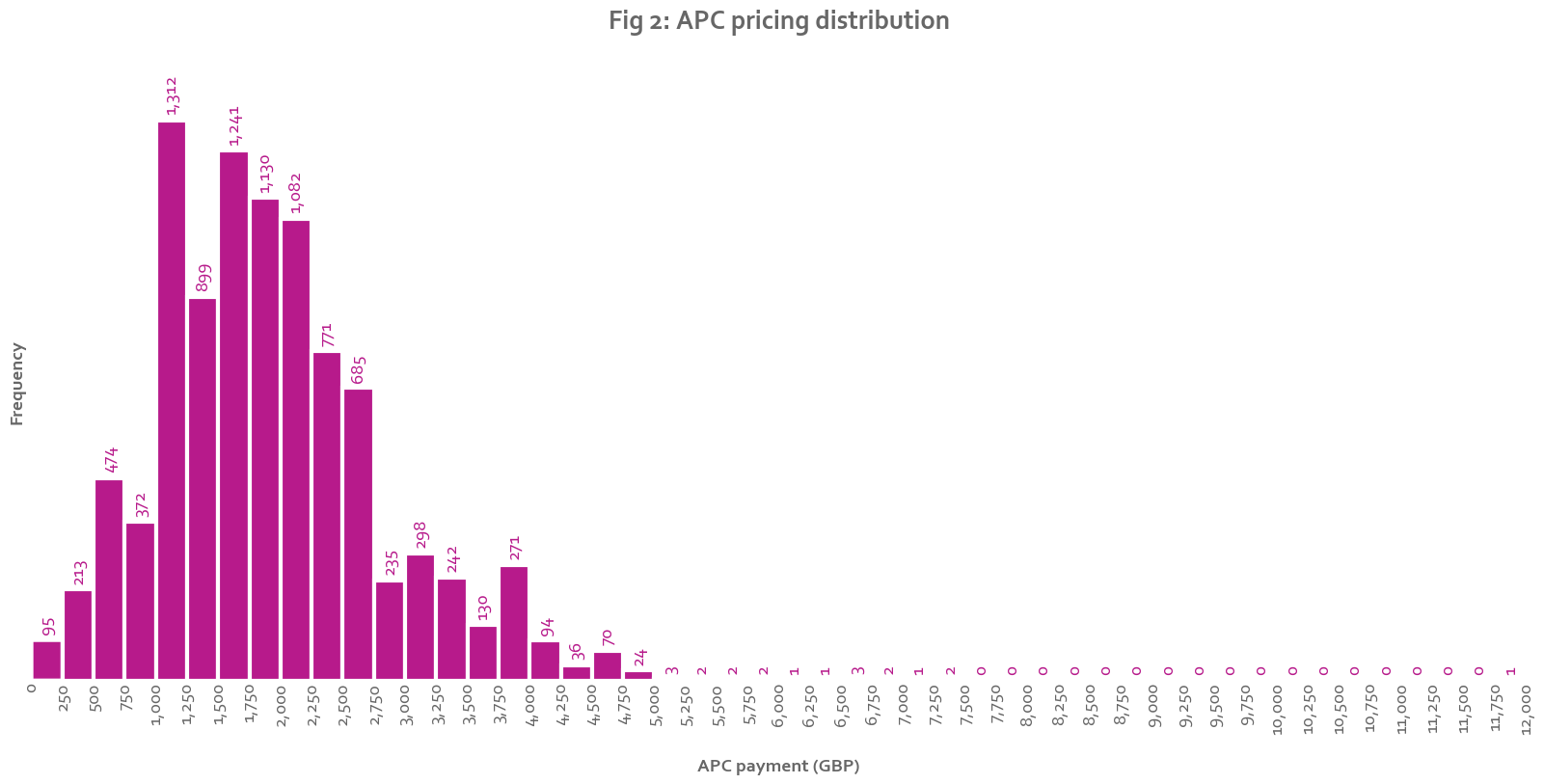Out:

APC prices are distributed around £1,800, with a long tail of more expensive APCs. Half the values are between £1,241 and £2,400.

# Trends in value and number¶

In :
graph_df0 = process_df(trend_df, groupby=year_col).reset_index()
title_end0 = "{} institutions".format(len(trend_insts))
graph_df1 = process_df(trend_df.loc[trend_df['Institution'] != 'UCL'], groupby=year_col).reset_index()
title_end1 = "{} institutions (outlier removed)".format(len(trend_insts)-1)

for graph_df,title_end in [(graph_df0, title_end0), (graph_df1, title_end1)]:
graph_df["Number growth"] = growth(graph_df["Number"])
graph_df["Expenditure growth"] = growth(graph_df["Expenditure (GBP)"])
graph_df["Average APC growth"] = growth(graph_df["Average APC (GBP)"])
# Draw the dual axis line-bar chart
x = range(len(graph_df))
y1 = graph_df["Number (thousands)"]
y2 = graph_df["Expenditure (GBP millions)"]
x_tick_labels = list(graph_df[year_col])
x_axis_label = "Year"
y1_label = "Number (thousands)"
y2_label = "Expenditure (GBP millions)"
y1_annotation = list(graph_df["Number growth"].apply(growthf))
y2_annotation = list(graph_df["Expenditure growth"].apply(growthf))
title = "Growth in APCs {}-{}, ".format(years, years[-1]) + title_end
line_bar_chart(x, y1, y2, title, x_tick_labels, x_axis_label, y1_label, y2_label, y1_annotation, y2_annotation)

for col in ["Number (thousands)", "Expenditure (GBP millions)", "Average APC (GBP)"]:
for graph_df,title_end in [(graph_df0, title_end0), (graph_df1, title_end1)]:
# Draw the bar chart (X values remain the same as above)
y = graph_df[col]
y_axis_label = col
if col == "Average APC (GBP)":
decimal_points = 0
else:
decimal_points = 1
labels = list(y.apply(lambda x: numf(x,decimal_points)))
title = "{} {}-{}, ".format(col, years, years[-1]) + title_end
bar_chart(x, y, title, x_tick_labels, x_axis_label, y_axis_label, labels)

# Save the data

save_df(graph_df, index=False)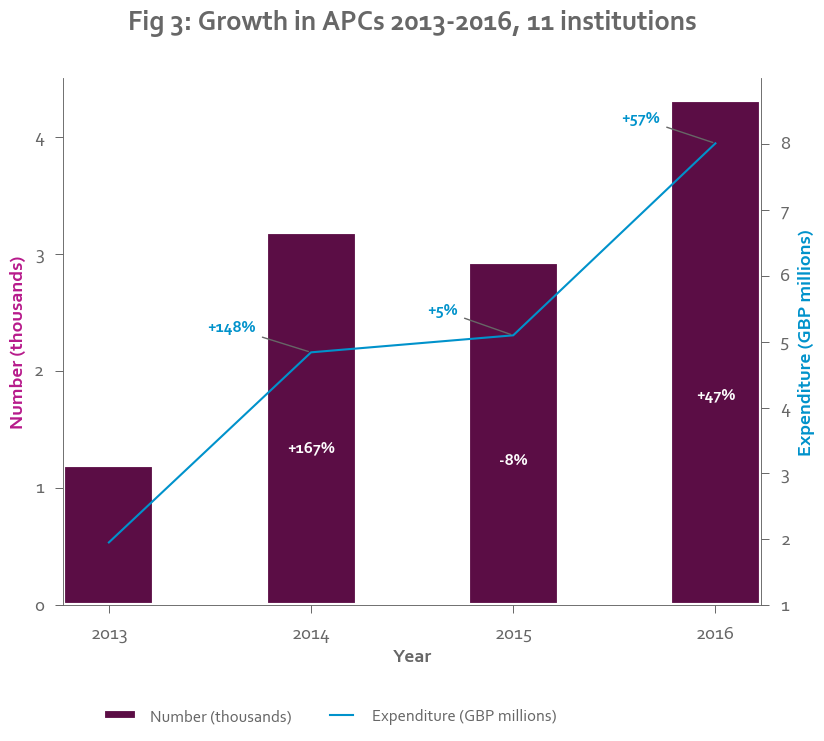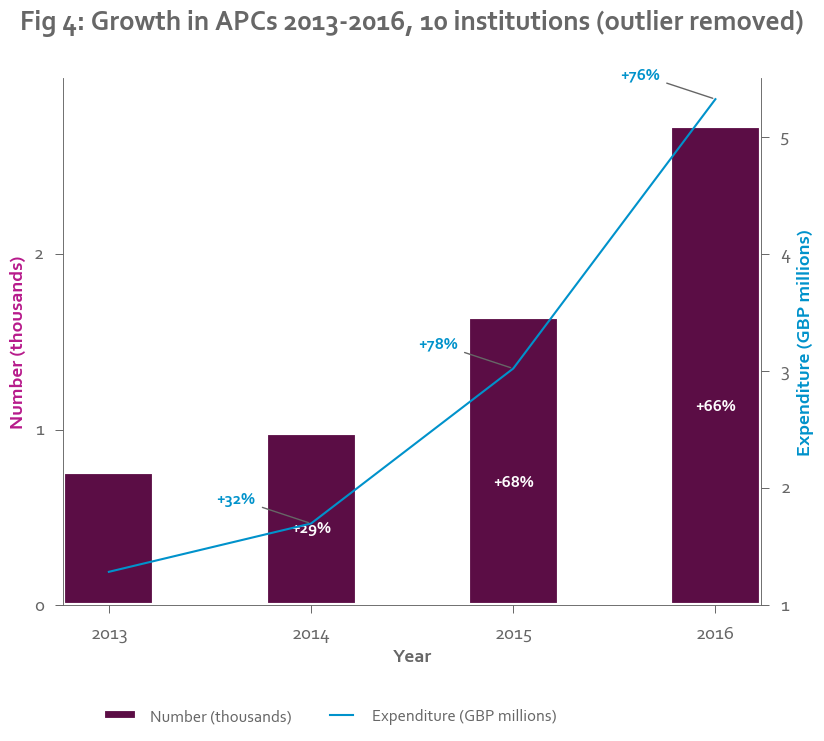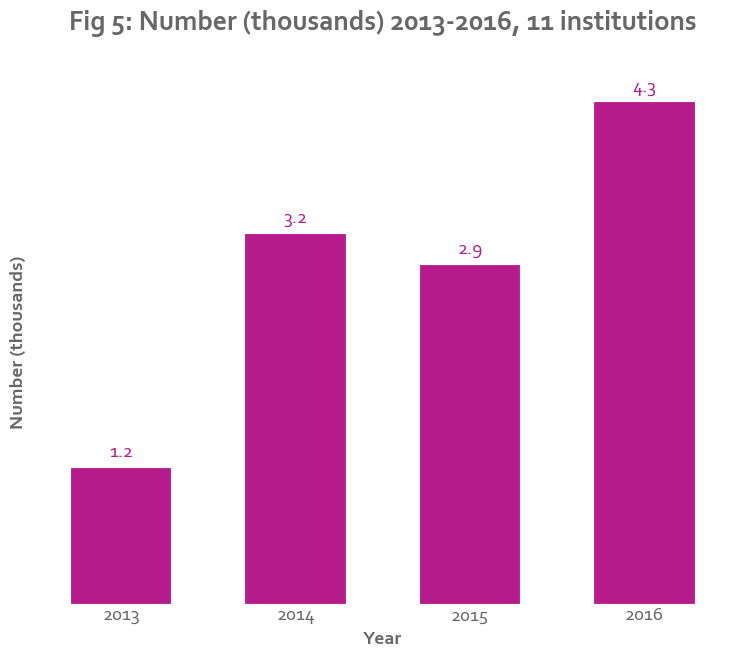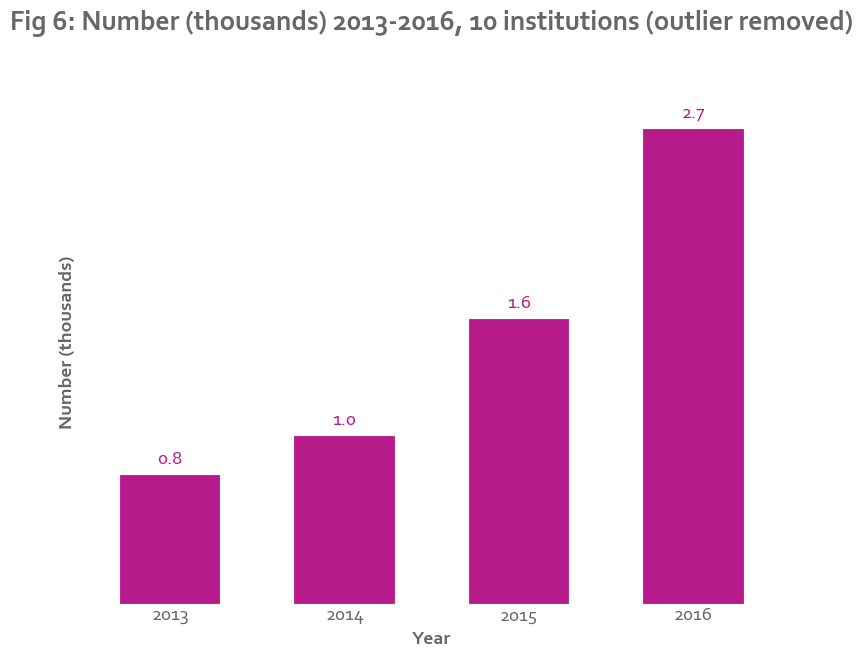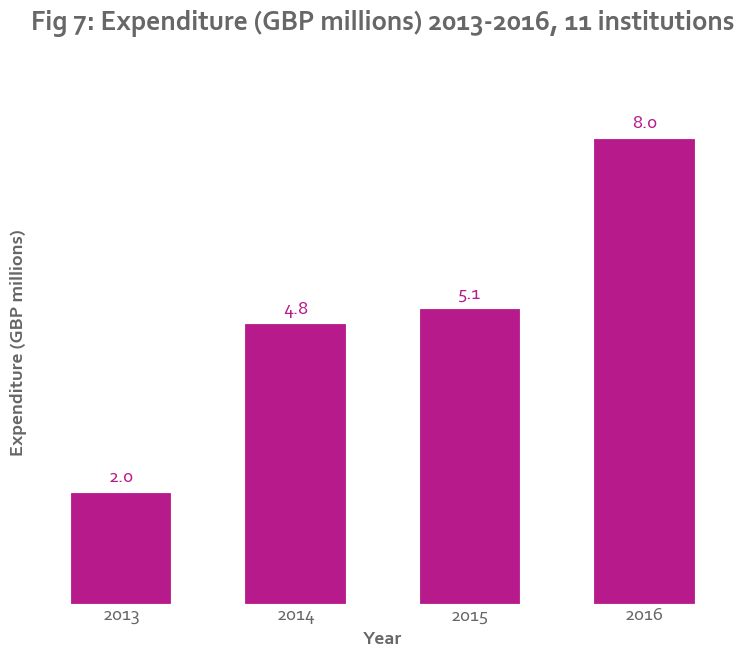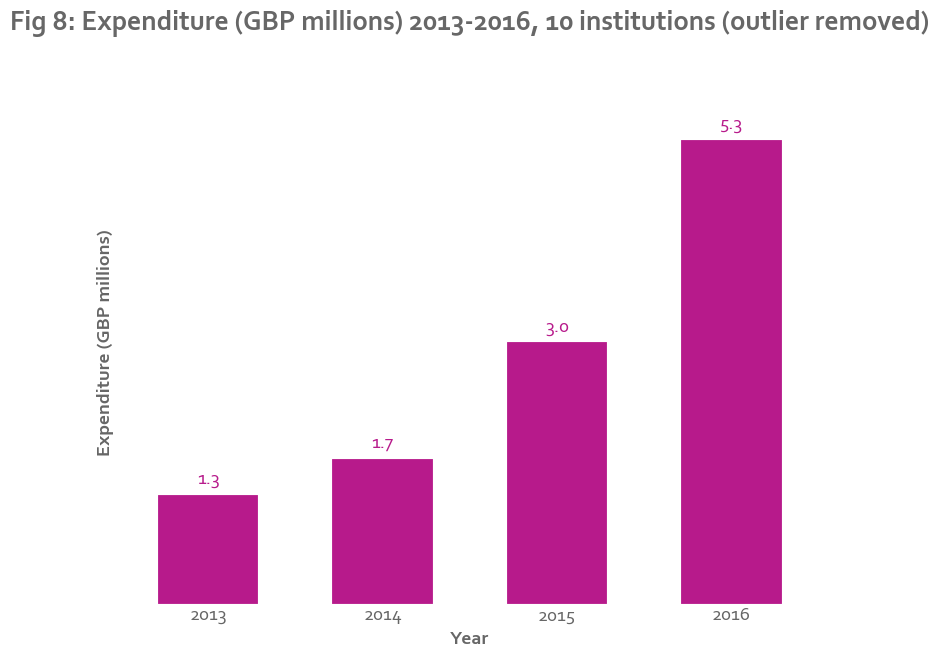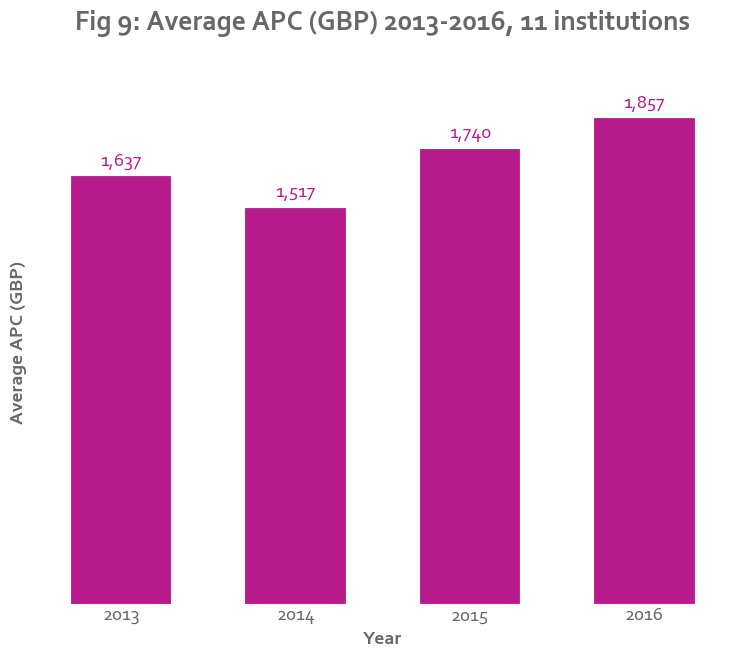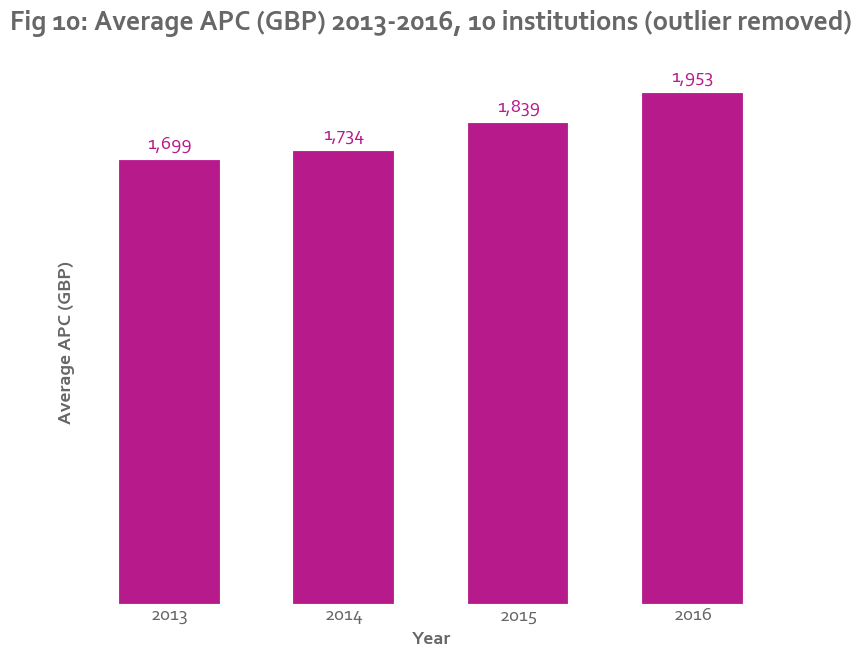APC number and expenditure have been growing steadily. There is a dip in 2015 that is mainly due to one large institution reducing its budget for APCs. With this institution removed, APC growth appears exponential.

# Breakdown by institution¶

In :
graph_df = process_df(current_df, 'Institution')
x = graph_df["Average APC (GBP)"]
title = "Average APCs paid by institution, {}".format(current_year)
xlabel = "Average APC paid"
binwidth = 100
histogram(x, title, xlabel, binwidth, labels=None)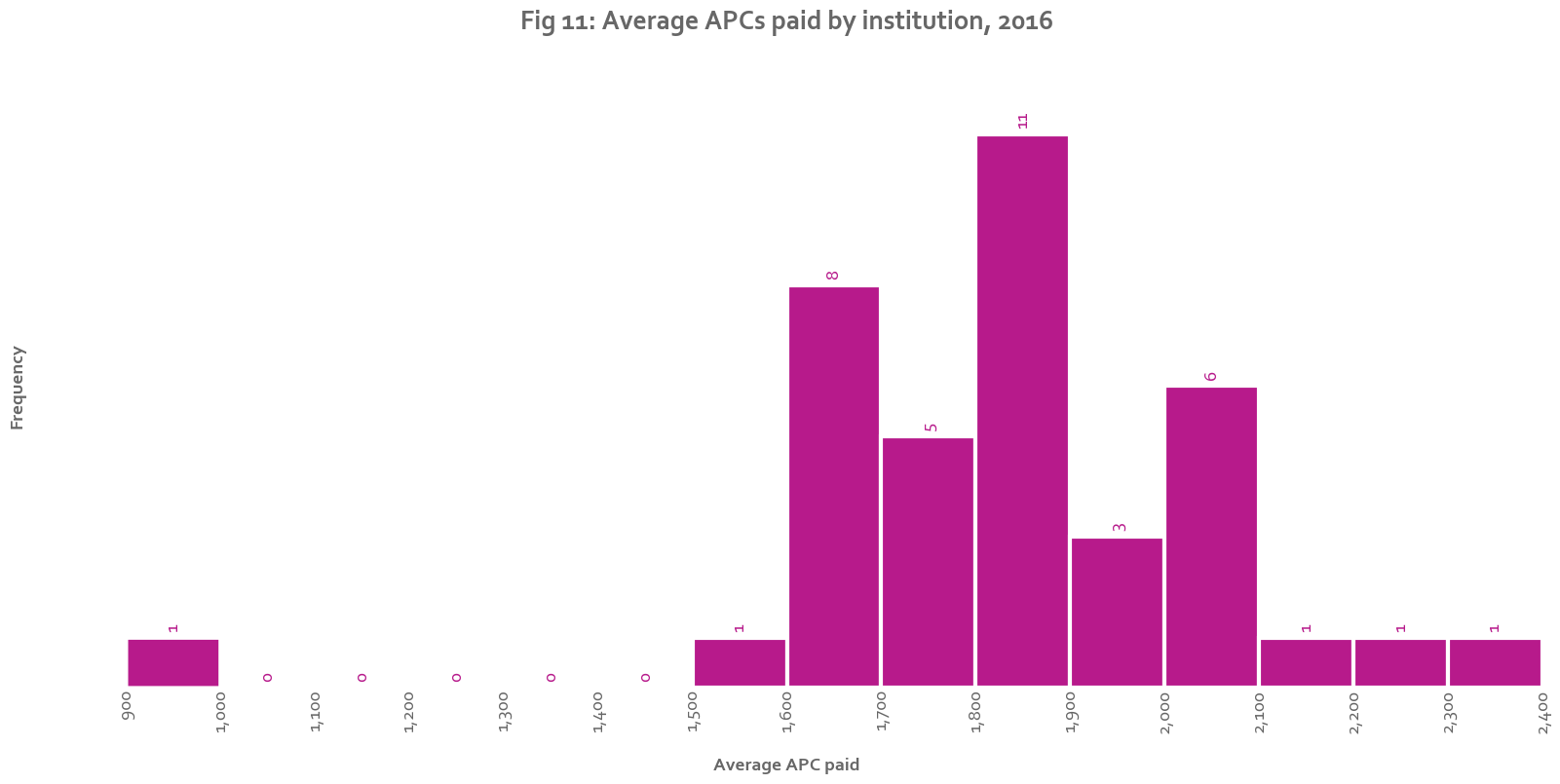Out:
(<matplotlib.figure.Figure at 0x150f7a20>,
<matplotlib.axes._subplots.AxesSubplot at 0x23bdbba8>)

The average APC paid by an institution varies. Most average within a few hundred pounds of the average price for an APC, but some pay much higher prices. This difference may be due to an institution's strength in disciplines that have a higher than average APC, or to a lack of use of offsetting deals and pre-payment agreements.

# Breakdown by publisher¶

## Current year¶

In :
# Separate out the top publishers
graph_df = cut_top(current_df, 'Publisher', top_pubs)
top_df = graph_df.loc[~graph_df["Publisher"].isin(["Other", "Total"])]
other_df = graph_df.loc[graph_df["Publisher"]=="Other"]
total_df = graph_df.loc[graph_df["Publisher"]=="Total"]

# Plot the bar charts
for col in ["Number", "Number including null- and zero-cost APCs", "Expenditure (GBP millions)", "Average APC (GBP)"]:
if col == "Average APC (GBP)":
append_value = "Total"
append_label = "Average across all publishers"
else:
append_value = "Other"
append_label = "All other publishers"

top_df = top_df.sort_values(by=col, ascending=True) # Sort in descending order
df = graph_df.loc[graph_df["Publisher"]==append_value].append(top_df).reset_index(drop=True) # Add "Other" or "Total" row to the bottom
df["Publisher"] = df["Publisher"].apply(lambda x: append_label if x == append_value else x) # Change the name to something easier to understand
if col == "Average APC (GBP)": # Re-sort the values
df = df.sort_values(by = col).reset_index(drop=True)
special_index = df.loc[df["Publisher"] == append_label].index # This index shoul dhave a different colour
else:
special_index = None
y = df.index
x = list(df[col])
title = "{} {}, {} institutions".format(col, years[-1], len(current_insts))
tick_labels =list(df["Publisher"])
if col == "Expenditure (GBP millions)":
decimal_places = 1
else:
decimal_places = 0
labels = map(lambda x: numf(x, decimal_places), x)
horizontal_bar_chart(y, x, title, tick_labels, labels=labels, special_index=special_index)

top_df = top_df.sort_values('Number including null- and zero-cost APCs')
df = other_df.append(top_df)
df["Publisher"] = df["Publisher"].apply(lambda x: 'All other publishers' if x == 'Other' else x) # Change the name to something easier to understand
y = range(len(df))
x0 = list(df['Number'])
x1 = list(df['Number including null- and zero-cost APCs'] - df['Number'])
x_labels = ["APCs with cost", "APCs with no cost or 0 GBP cost" ]
tick_labels = list(df['Publisher'])
colours = [plot_colours["purple"], plot_colours["dark purple"]]
stacked_horizontal_bar_chart(y,
[x0,x1],
'Number of APCs with and without cost data, {} institutions'.format(len(current_insts)),
tick_labels=tick_labels,
x_labels=x_labels,
x_axis_label="Number",
colours=colours )

# Calculations for the text below
top_df = top_df.sort_values(by="Expenditure (GBP)", ascending=False).reset_index(drop=True) # Sort by expenditure
top_pub_by_exp = top_df["Publisher"] # Top publisher by expenditure
top_pub_exp = top_df["Expenditure (GBP)"] # Amount spent with that publisher
total_exp = total_df["Expenditure (GBP)"] # Total expenditure across all publishers
top_pub_market_share = top_pub_exp / total_exp # Market share of top publisher
second_pub_by_exp = top_df["Publisher"] # Second to top publisher
second_pub_exp = top_df["Expenditure (GBP)"] # Amount spent with second publisher
top_second_exp_comparison = top_pub_exp / second_pub_exp # Comparison between the two
top_10_market_share = top_df["Expenditure (GBP)"].sum() / total_exp
num_other_pubs = master_df["Publisher"].nunique() - len(top_pubs)

top_df = top_df.sort_values(by="Number including null- and zero-cost APCs", ascending=False).reset_index(drop=True) # Sort by number including £0 APCs
top_pub_by_num_w_zero = top_df["Publisher"] # Top publisher by number
top_pub_num_w_zero = top_df["Number including null- and zero-cost APCs"] # Number of APCs with that publisher
total_num_w_zero = total_df["Number including null- and zero-cost APCs"] # Total number
top_pub_number_share = float(top_pub_num_w_zero) / total_num_w_zero # Top pub share of the number
top_pub_by_exp_num_w_zeros = top_df.loc[top_df["Publisher"] == top_pub_by_exp]["Number including null- and zero-cost APCs"] # Number of APCs with the top pub
top_num_w_zero_comparison = float(top_pub_num_w_zero / top_pub_by_exp_num_w_zeros) # Comparison between the number of APCs spent with top pub by number and by expenditure

top_df = top_df.sort_values(by="Average APC (GBP)", ascending=False).reset_index(drop=True)  # Sort by average APC
top_pub_by_avg = top_df["Publisher"] # Major publisher with highest average APC
top_pub_avg_apc = top_df["Average APC (GBP)"] #  That publisher's average APC
total_avg_apc = total_df["Average APC (GBP)"] # The global average APC
top_avg_comparison = top_pub_avg_apc / total_avg_apc # Comparison between the two

save_df(graph_df)

HTML('''
<p>
A few large publishers capture most of the market share. The top {} publishers make up {} of the market,
with the remaining {} publishers capturing the rest of the long tail. {} makes up {}
of APC expenditure, and captures {} times as much revenue from APCs than its
next nearest rival, {}.
</p>
'''.format(len(top_pubs), pctf(top_10_market_share), num_other_pubs, top_pub_by_exp, pctf(top_pub_market_share), numf(top_second_exp_comparison, 2), second_pub_by_exp )
+
'''
<p>
However, this revenue does not include APCs that were paid for from offset deals,where the amount of the APC is
unknown or unclear. If we look at the publishers in terms of the number of APCs paid (including APCs marked as &pound0
or without a value), the publisher with the most APCs is {}, with {} times more APCs than {}, or {} of the total number.

</p>
'''.format(top_pub_by_num_w_zero, numf(top_num_w_zero_comparison, 2), top_pub_by_exp, pctf(top_pub_number_share) )
+
'''
<p>
APC pricing varies significantly between publishers. The highest average APC charged by a major publisher is
&pound{} charged by {}, much higher than the average APC of &pound{}.
</p>
'''.format(numf(top_pub_avg_apc, 0),top_pub_by_avg,numf(total_avg_apc, 0) )

)